5G - Massive MIMO                                                          Home : www.sharetechnote.com Massive MIMO   As the name implies, Massive MIMO is an antenna array system using Massive Amount of Antenna. It is also called Large Scale MIMO. Then you may ask "How many Antenna is required to be called 'Massive (Large Scale) MIMO' ?". The answer may be different depending on how you design the reciever algorithm (equalizer design), but assuming that we use the simplest (the most primitive) reciever design, we may need over 300 antenna. If you think this is too big number, you may decrease the number by applying a little bit smarter reciever (equalizer) algorithm. In conventional LTE using a normal MIMO, the maximum number of antenna in MIMO as of now (Mar 2015) is 8 x 2 or 4 x 4.       Why Massive MIMO ?   I think the main reason for Massive MIMO for 5G is 'there is no other choice'. It is highly likely that we will use very high frequency (mm Wave) signal in 5G. High frequency mean that the size of single antenna will be very small and the aperture (the area for receiving energy) will be very small. To overcome this small aperture on reciever side at high frequency, we need to use a large number of transmission antenna. This would be the main reason, but once we adopt the Massive MIMO technology, we can enjoy some other advantages coming from using a large array antenna that will be described later.   Now let's look more into the meaning of 'there is no other choice'. I will describe on this aspect based on WNCG Prof. Robert Heath on Millimeter Wave MIMO Communication (I recommend you to look into the presentation on YouTube).   Let's assume a situation where we have one transmission antenna and one reciever antenna placed with distance R as illustrated below.If the transmission antenna transmit signal with the power of Ptx, what would be the received signal power ? If we assume the ideal condition, the received power can be represented as follows. This would be very familiar form that you have known from high school physics, so called squared inverse rule. The received power is decreased in proportion to the square of the distance from the transmission antenna. For example, if the distance gets two times farther away, the received power gets decreased by 4 times.It sounds very simple. This ideal equation does not contain any parameter about frequency or the gain of the reciever antenna. It means the received signal power is not influenced by signal frequency or reciever antenna gain. But we know this is not true from our common sense in radio communication. In real life, the received signal power IS affected by the frequency (wave length) and reciever antenna gain. If we improve the mathematical model to include the frequency (wave length) and reciever antenna gain, the model can be described as shown below. According to this equation, the recieved power is in proprtion to the square of the wavelength. For example, if we assume the antenna gain does not changes, the frequency gets increased by 2 times (this mean that the wavelength gets shorten by 2 times), the recieved power gets decreased by 4 times.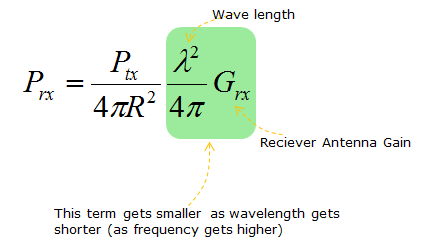As I mentioned before, we will use much higher frequency (meaning much shorter wavelength) signal in 5G. It means the received power will be much lower than in current communication system. For example, if we use 2 Ghz frequency in current communication and we will use 20 Ghz frequency in 5G, the wavelength in 20 Ghz is 10 times shorter than the wavelength of 2 Ghz. It means the received power at 20 Ghz will be 100 times lower than the received power at 2 Ghz.   In reality, the situation gets even more complicated because not only the reciever antenna gain but also transmission antenna gain plays role as well. If we add the transmission antenna gain into the equation, it would become as shown below.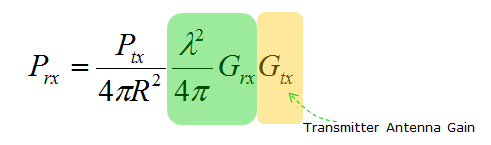Now let's think of how we can overcome the drastic received power reduction at high frequency. In other words, the question is 'how we can make Prx larger ?'. Mathematically it is simple. You can get larger Prx by setting the parameters as follows. i) Increase Ptx (Transmiter Power) ii) Decrease the distance between the transmiter and reciever antenna. iii) Increase wavelength (use low frequency) iv) increase reciever antenna gain v) increase transmitter antenna gain. In real life, can we use all of these options ? The answer is 'No'. Let's look at each of these option one by one and think which one can be applicable in real life.   Option i) can be doable to a certain degree, but we cannot increase the transmitter power as much as we want. Option ii) cannot be the solution since we cannot change the distance as we like. Option iii) cannot be the solution. Once we (standard organization and each  Network Operator) decided to use a certain frequency, we have to follow it. We cannot change as we like. Option iv) and Option v) can be a doable solution. It may not be easy to increase the antenna gain, but at least nobody (standard organization, Network Operator) does not prevent us from trying to increase the antenna gain.   Then how can we increase the antenna gain ? We may increase the antenna gain by design (e.g, shape, material etc), but the amount of gain improvement by design cannot be as large as to compensate the huge amount of the power reduction cause by the increased frequency. Almost the only way in this case would be to increase the number of antenna and it is the major motivation of using Massive MIMO as illustrated below.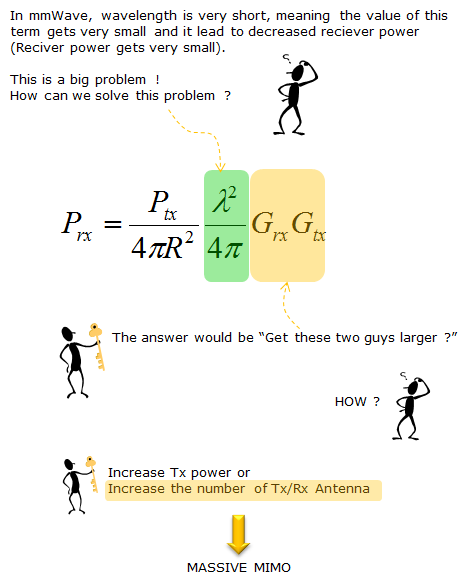In addition to increasing the received power, Massive MIMO provides several other advantages as well.   According to "Massive MIMO for Next Generation Wireless Systems" (), the potential (advantage) of Massive MIMO is described as follows; Massive MIMO can increase the capacity 10 times or more and simultaneously, improve the radiated engergy-efficiency in the order of 100 times. Massive MIMO can be built with inexpensive, low-power consumption Massive MIMO enables a significant reduction of latency on the air interface (due to robustness against fading) Massive MIMO simplifies the multiple-access layer Massive MIMO increases the robustness both to unintended man-made interference and to intentional jamming.     Spatial Focus with More Antenna   Following is based on Scaling up MIMO : Opportunities and Challenges with Very Large Arrays   Following is to illustrate how large antenna arrays can focus the electromagnetic field to a certain geographic point. This specific case shows the resulting normalized field strength. (This example huses TRBF(Time-Reversal BeamForming) with MF precodings.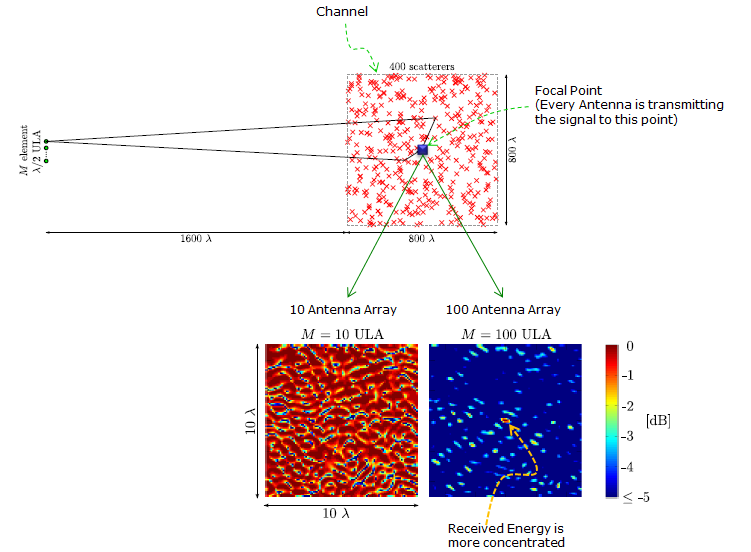This result shows i) the field strength can be focused to a point rather than in a certain direction ii) more antenna improve the ability to focus engergy to a certain point With enough many antennas and favorable propagaton i) TRBF focus power and yield a high spectral efficientcy through spatial multiplexing to many terminals ii) TRBF also reduce (or completely elminate in ideal case) inter-symbol interference, meaning that we could dispense with OFDM and its redundant cyclic prefix.   Process being done by each base station antenna i) convolve the data sequence intended for the k-th terminal with the conjugated, time-reversed version of his estimate for the channel impulse response to the k-th terminal ii) sum the K convolutions iii) feed that sum into the antenna     Channel Model for Point to Point MIMO   Following is based on Scaling up MIMO : Opportunities and Challenges with Very Large Arrays   Channel Model : Following is the brief mathematicl description of channel model for MIMO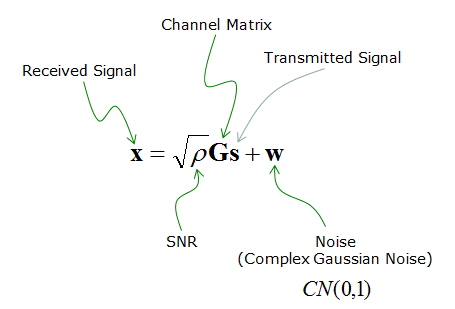Achievable Rate : Following is the mathematical model expressing the achievable data rate. This is generic form and you see all the factors (e.g, Number of Tx, Rx Antenna, SNR, Channel Matrix) in the equation. But depending on the situation, the dominant factors gets different and you can approximate this generic for to various other (simpler) form.Another way of expressing this formular is as follows. This equation represents the same thing using the diagonal numbers of Singular Matrix obtained by SVD. (SVD is pretty important mathematical tools for MIMO modeling. If you are not familiar with this, see SVD page for understanding the concept and refer to LTE MIMO page to see an application)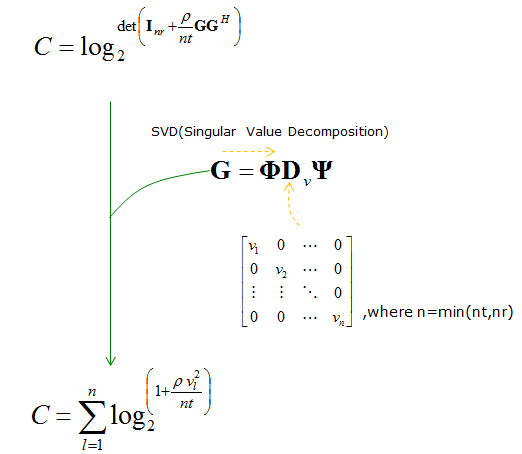When SNR is very low as in cell edge, this equation can be approximated as follows. (How the orginal equation can  be approximated like this is described  in detail in this paper. You may need to look for another paper if you are really understand the details.)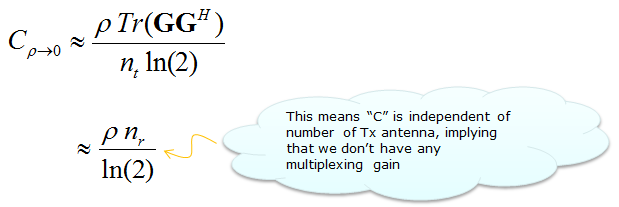When the number of transmitter antenna gets very large comparing to the number of reciever antenna, following term can be approximated to an Identity Matrix. (How this approximation can be derived is not described in this paper)Using this approximation, you can rewrite the original equation as shown below.When the number of reciever antenna gets very large comparing to the number of transmitter antenna, the original equation can be expressed as follows.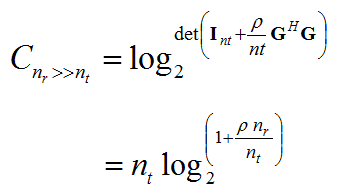Channel Model for Multi User MIMO   Following is based on Scaling up MIMO : Opportunities and Challenges with Very Large Arrays, this is based on TDD system. It is assumed that a BTS has large number of Antenna and they are communicating with multiple UEs and each UE has only one antenna.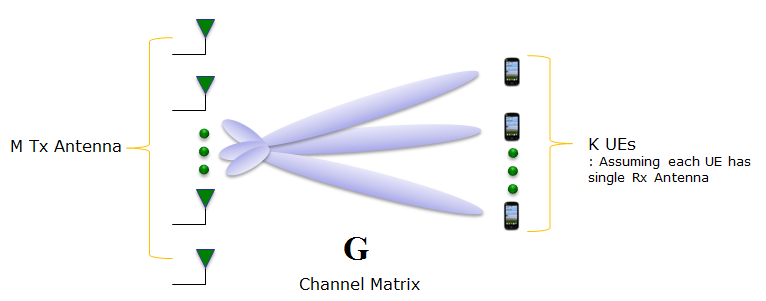The channel matrix can be represented as follows.Reverse Link   The communication model for reverse path (from UE to BTS) is represented as shown below.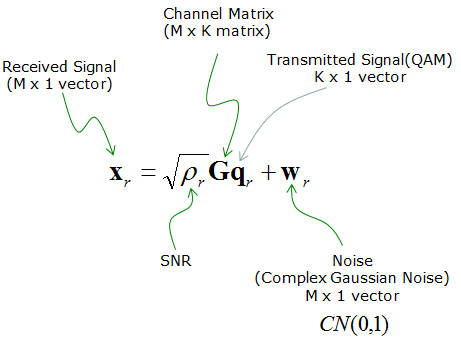BTS can process the received data as following equation.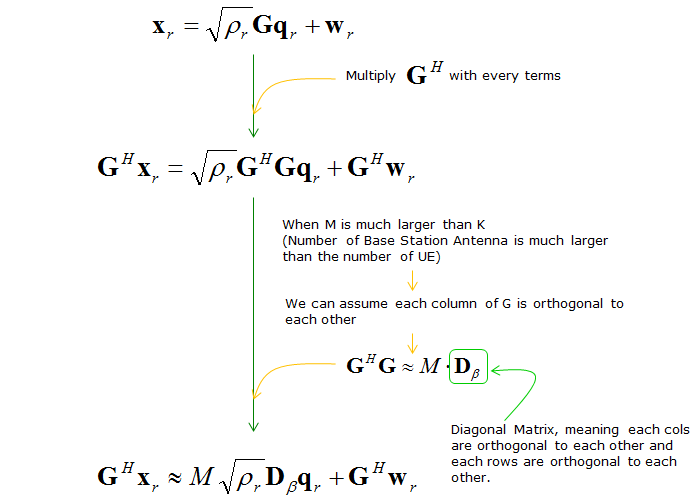Capacity for this channel can be described as follows.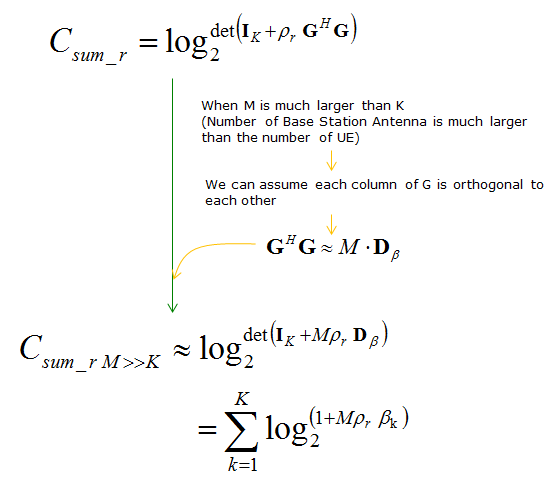Forward Link   Communication model for Forward Link (from BTS to UE) can be described as follows.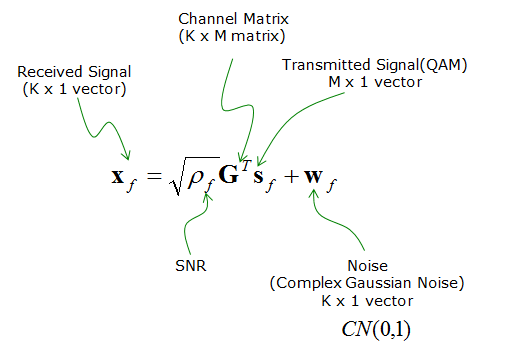The reciever (UE) can process the received data according to following equation.The capacity for this link can be described as follows.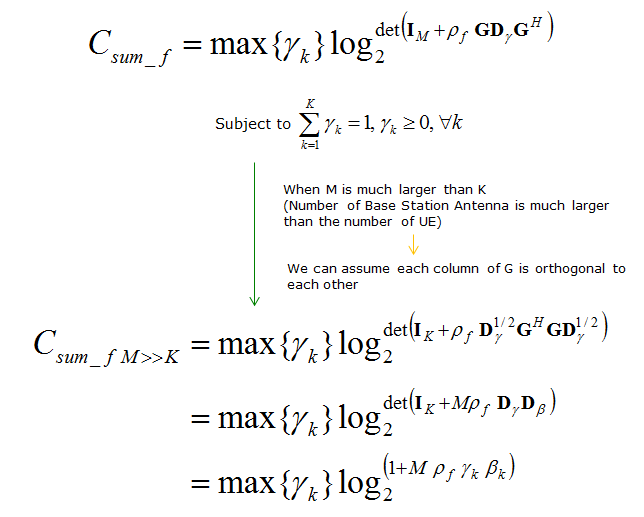< Channel Model : Massive MIMO based on Many Small Cells >   Followings are based on Massive MIMO and Small Cells : Improving Energy Efficiency by Optimal Soft-Cell Coordination ().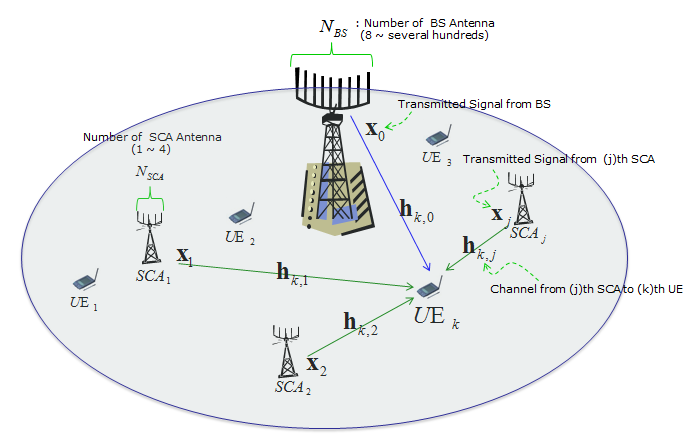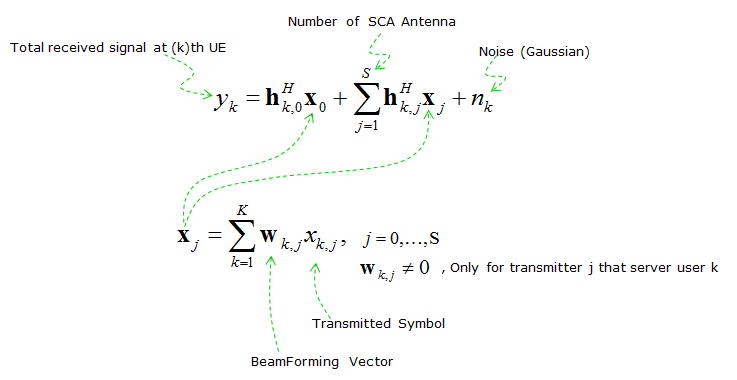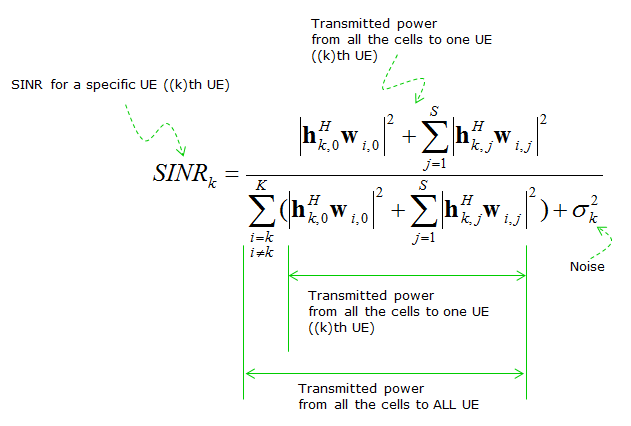Reference    GFDM Interference Cancellation for Flexible Cognitive Radio PHY Design R. Datta, N. Michailow, M. Lentmaier and G. Fettweis Vodafone Chair Mobile Communications Systems, Dresden University of Technology, 01069 Dresden, Germany Email:[rohit.datta, nicola.michailow, michael.lentmaier, fettweis]@ifn.et.tu-dresden.de  5G NOW. D3.1 5G Waveform Candidate Selection    Massive MIMO and Small Cells : Improving Energy Efficiency by Optimal Soft-Cell Coordination Emil Bjornson, Marios Kountouris and Merouane Debbah Alcatel-Lucent Chair on Flexible Radio, SUPELEC, Gif-sur-Yvette, France Department of Telecommunications, SUPELEC, Gif-sur-Yvette, France ACCESS Linnaeus Center, Signal Processing Lab, KTH Royal Institue of Technology, Stockholm, Sweden      Massive MIMO for Next Generation Wireless Systems Erik G. Larson, ISY, Linkoping University, Sweden Ove Edfors, Lund University, Sweden Fredrik Tufvesson, Lund University, Sweden Thomas L. Marzetta, Bell Labs, Alcatel-Lucent, USA    Scaling up MIMO : Opportunities and Challenges with Very Large Arrays Fredrik Rusek, Dept. of Electrical and Information Technology, Lund University, Lund, Sweden Daniel Persson, Dept. of Electrical Engineering (ISY), Linkoping University, Sweden Buon Kiong Lau, Dept. of Electrical and Information Technology, Lund University, Lund, Sweden Erik G. Larsson, Dept. of Electrical Engineering (ISY), Linkoping University, Sweden Thomas L. Marzetta, Bell Laboratories, Alcatel-Lucent, Murray Hill, NJ Ove Edfors, Dept. of Electrical and Information Technology, Lund University, Lund, Sweden Fredrik Tufvesson, Dept. of Electrical and Information Technology, Lund University, Lund, Sweden     Fundamentals of Massive MIMO (YouTube)